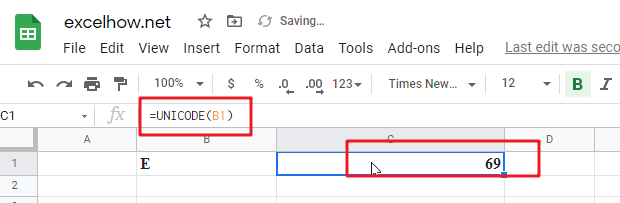# ExcelHow

This post will guide you how to use Google Sheets UNICODE function with syntax and examples.

## Description

The Google Sheets UNICODE function returns the decimal Unicode value of the first character of the text.

The UNICODE function can be used to return a number corresponding to a Unicode character in google sheets.  The purpose of this function is to get number from a Unicode character and its returned value is a decimal number.

The UNICODE function is a build-in function in Google Sheets and it is categorized as a Text Function.

## Syntax

The syntax of the UNICODE function is as below:

= UNICODE(text)

Where the UNICODE function argument is:

• text -This is a required argument. The string containing the character to be converted

Note:

• Unicode can be implemented by different character encodings. The Unicode standard defines Unicode Transformation Formats (UTF): UTF-8, UTF-16, and UTF-32, and several other encodings.
• The UNICODE function only words properly if the input text string is not empty and the first character has a Unicode representation.
• Number can be passed directly into the UNICODE function, returning as a string.

## Google Sheets UNICODE Function Examples

The below examples will show you how to use google sheets UNICODE function to convert a Unicode character to a number.

#1 To get a number from a Unicode character in cell B1, just using the following formula:

`=UNICODE(B1)  //it returns E`Note: you can use the UNICHAR function to perform the opposite conversion to return the Unicode character for a number.